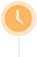• 23:34 三角形
中国吉林网
• 23:23 三角形
• 23:15 三角形
• 22:16 三角形
中国吉林网
• 21:31 三角形
• 21:26 三角形
• 21:13 三角形
中国吉林网
• 20:21 三角形
• 18:46 三角形
中国吉林网
• 18:35 三角形
• 15:50 三角形
中国吉林网
• 15:48 三角形
• 15:44 三角形
中国吉林网
• 15:27 三角形
• 15:00 三角形
• 14:58 三角形
中国吉林网
• 14:51 三角形
中国吉林网
• 14:42 三角形
中国吉林网
• 12:09 三角形
• 10:27 三角形
中国吉林网
• 09:50 三角形
• 09:25 三角形
• 22:02 三角形
• 21:55 三角形
• 21:43 三角形
• 17:11 三角形
中国吉林网
• 16:40 三角形
• 16:31 三角形
• 16:31 三角形
• 16:27 三角形
• 13:23 三角形
• 12:20 三角形
• 11:36 三角形
• 11:07 三角形
• 10:49 三角形
• 10:11 三角形
• 08:00 三角形
• 00:19 三角形
• 22:13 三角形
• 18:09 三角形
中国吉林网
• 18:03 三角形
中国吉林网
• 16:25 三角形
中国吉林网
• 14:41 三角形
• 12:35 三角形
中国吉林网
• 12:29 三角形
中国吉林网
• 11:27 三角形
• 09:59 三角形
• 09:57 三角形
• 08:36 三角形
• 08:06 三角形
中国吉林网• 三角形
中国吉林网
• 三角形
• 三角形
• 三角形
中国吉林网
• 三角形
• 三角形
• 三角形
中国吉林网
• 三角形
• 三角形
中国吉林网
• 三角形
• 三角形
中国吉林网
• 三角形
• 三角形
中国吉林网
• 三角形
• 三角形
• 三角形
中国吉林网
• 三角形
中国吉林网
• 三角形
中国吉林网
• 三角形
• 三角形
中国吉林网
• 三角形
• 三角形
• 三角形
• 三角形
• 三角形
• 三角形
中国吉林网
• 三角形
• 三角形
• 三角形
• 三角形
• 三角形
• 三角形
• 三角形
• 三角形
• 三角形
• 三角形
• 三角形
• 三角形
• 三角形
• 三角形
中国吉林网
• 三角形
中国吉林网
• 三角形
中国吉林网
• 三角形
• 三角形
中国吉林网
• 三角形
中国吉林网
• 三角形
• 三角形
• 三角形
• 三角形
• 三角形
中国吉林网
• 三角形
中国吉林网
• 三角形
• 三角形
• 三角形
中国吉林网
• 三角形
中国吉林网
• 三角形
• 三角形
中国吉林网
• 三角形
• 三角形
中国吉林网
• 三角形
• 三角形
中国吉林网
• 三角形
• 三角形
• 三角形
中国吉林网
• 三角形
中国吉林网
• 三角形
中国吉林网
• 三角形
中国吉林网
• 三角形
• 三角形
• 三角形
• 三角形
• 三角形
• 三角形
• 三角形
中国吉林网
• 三角形
• 三角形
• 三角形
中国吉林网
• 三角形
中国吉林网
• 三角形
• 三角形
• 三角形
• 三角形
中国吉林网
• 三角形
• 三角形
• 三角形
• 三角形
中国吉林网
• 三角形
• 三角形
中国吉林网
• 三角形
• 三角形
中国吉林网
• 三角形
• 三角形
• 三角形
• 三角形
• 三角形
• 三角形
• 三角形
• 三角形
中国吉林网
• 三角形
中国吉林网
• 三角形
中国吉林网
• 三角形
中国彩虹网
• 三角形
中国吉林网
• 三角形
• 三角形
• 三角形
• 三角形
中国吉林网
• 三角形
• 三角形
• 三角形
中国吉林网
• 三角形
• 三角形
• 三角形
中国吉林网
• 三角形
• 三角形
• 三角形
• 三角形
中国吉林网
• 三角形
• 三角形
中国吉林网
• 三角形
• 三角形
• 三角形
• 三角形
中国吉林网
• 三角形
中国吉林网
• 三角形
• 三角形
中国吉林网
• 三角形
中国吉林网
• 三角形
• 三角形
• 三角形
• 三角形
中国吉林网
• 三角形
• 三角形
中国吉林网
• 三角形
中国吉林网
• 三角形
中国吉林网
• 三角形
• 三角形
• 三角形
• 三角形
• 三角形
• 三角形
中国吉林网
• 三角形
• 三角形
• 三角形
• 三角形
• 三角形
• 三角形
• 三角形
• 三角形
• 三角形
中国吉林网
• 三角形